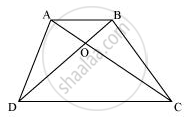# Diagonals AC and BD of a quadrilateral ABCD intersect at O in such a way that ar (AOD) = ar (BOC). Prove that ABCD is a trapezium. - Mathematics

Diagonals AC and BD of a quadrilateral ABCD intersect at O in such a way that ar (AOD) = ar (BOC). Prove that ABCD is a trapezium.

#### SolutionIt is given that

Area (ΔAOD) = Area (ΔBOC)

Area (ΔAOD) + Area (ΔAOB) = Area (ΔBOC) + Area (ΔAOB)

We know that triangles on the same base having areas equal to each other lie between the same parallels.

Therefore, these triangles, ΔADB and ΔACB, are lying between the same parallels.

i.e., AB || CD

Therefore, ABCD is a trapezium.

Concept: Corollary: Triangles on the same base and between the same parallels are equal in area.
Is there an error in this question or solution?

#### APPEARS IN

NCERT Class 9 Maths
Chapter 9 Areas of Parallelograms and Triangles
Exercise 9.3 | Q 15 | Page 164